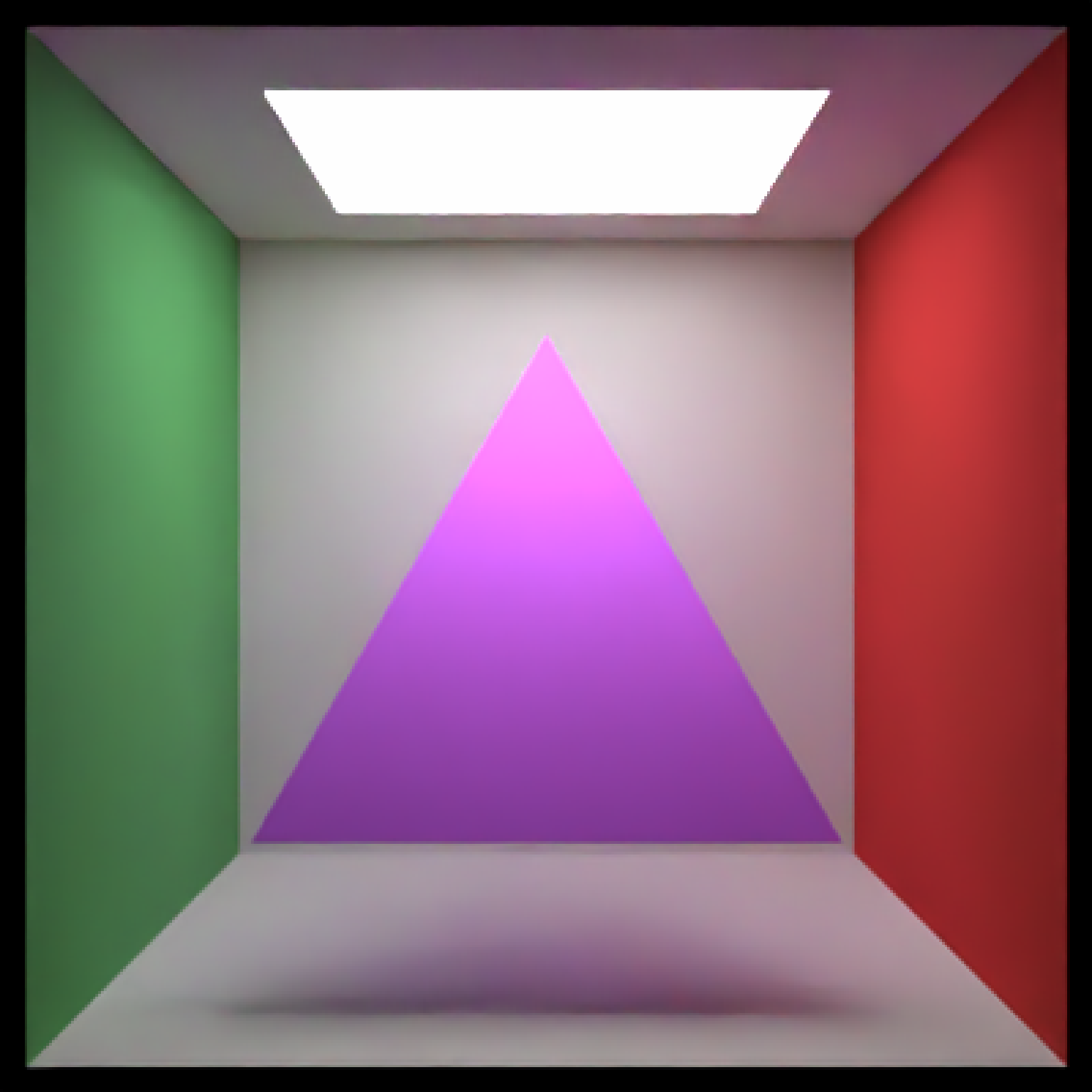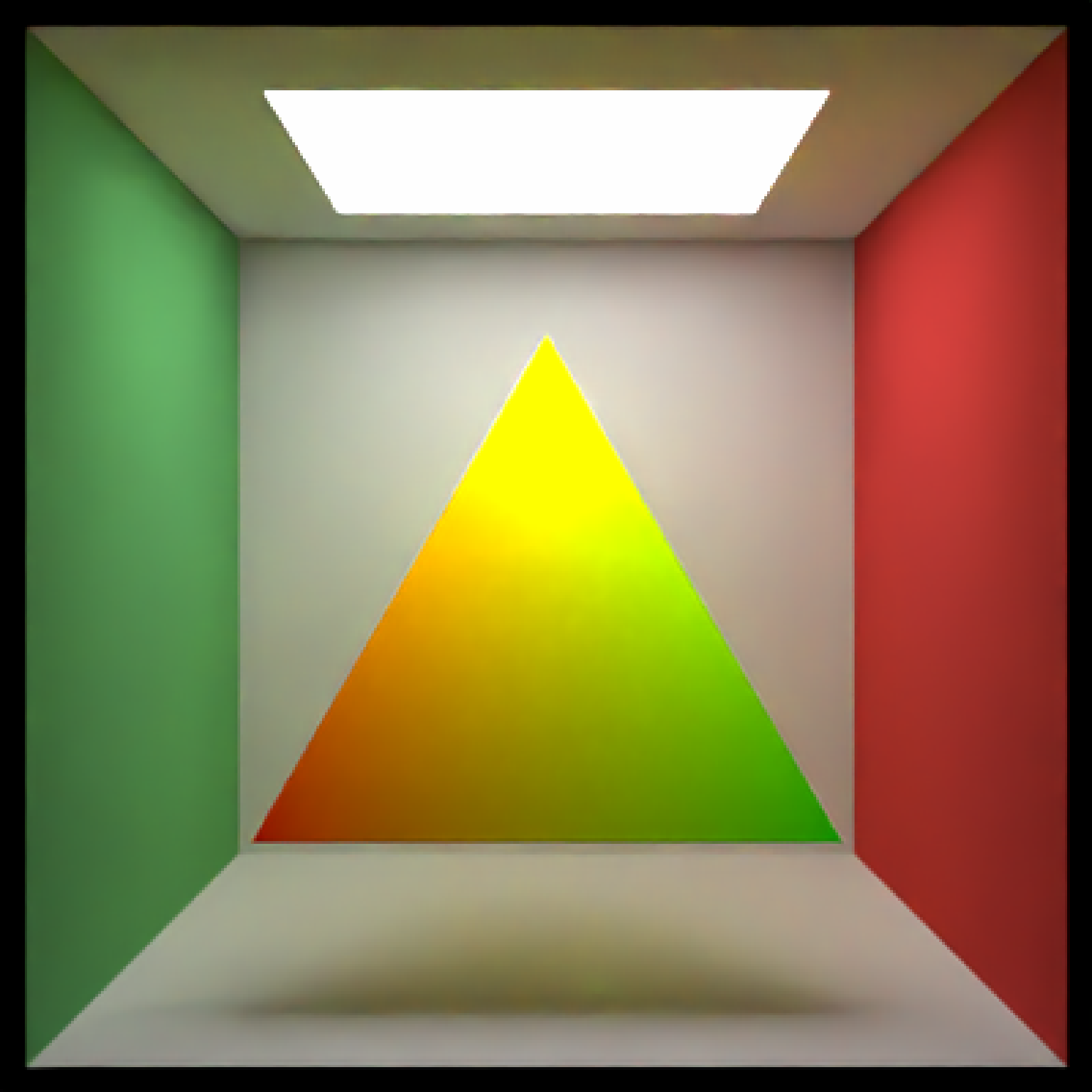Triangle Object

triangle(
v1 = c(1, 0, 0),
v2 = c(0, 1, 0),
v3 = c(-1, 0, 0),
n1 = rep(NA, 3),
n2 = rep(NA, 3),
n3 = rep(NA, 3),
color1 = rep(NA, 3),
color2 = rep(NA, 3),
color3 = rep(NA, 3),
material = diffuse(),
angle = c(0, 0, 0),
order_rotation = c(1, 2, 3),
flipped = FALSE,
reversed = FALSE,
scale = c(1, 1, 1)
)

## Arguments

v1 Default c(1, 0, 0). Length-3 vector indicating the x, y, and z coordinate of the first triangle vertex. Default c(0, 1, 0). Length-3 vector indicating the x, y, and z coordinate of the second triangle vertex. Default c(-1, 0, 0). Length-3 vector indicating the x, y, and z coordinate of the third triangle vertex. Default NA. Length-3 vector indicating the normal vector associated with the first triangle vertex. Default NA. Length-3 vector indicating the normal vector associated with the second triangle vertex. Default NA. Length-3 vector indicating the normal vector associated with the third triangle vertex. Default NA. Length-3 vector or string indicating the color associated with the first triangle vertex. If NA but other vertices specified, color inherits from material. Default NA. Length-3 vector or string indicating the color associated with the second triangle vertex. If NA but other vertices specified, color inherits from material. Default NA. Length-3 vector or string indicating the color associated with the third triangle vertex. If NA but other vertices specified, color inherits from material. Default diffuse.The material, called from one of the material functions diffuse, metal, or dielectric. Default c(0, 0, 0). Angle of rotation around the x, y, and z axes, applied in the order specified in order_rotation. Default c(1, 2, 3). The order to apply the rotations, referring to "x", "y", and "z". Default FALSE. Whether to flip the normals. Default FALSE. Similar to the flipped argument, but this reverses the handedness of the triangle so it will be oriented in the opposite direction. Default c(1, 1, 1). Scale transformation in the x, y, and z directions. If this is a single value, number, the object will be scaled uniformly. Note: emissive objects may not currently function correctly when scaled.

## Value

Single row of a tibble describing the XZ plane in the scene.

## Examples

#Generate a triangle in the Cornell box.
# \donttest{
generate_cornell() %>%
add_object(triangle(v1 = c(100, 100, 100), v2 = c(555/2, 455, 455), v3 = c(455, 100, 100),
material = diffuse(color = "purple"))) %>%
render_scene(lookfrom = c(278, 278, -800) ,lookat = c(278, 278, 0), fov = 40,
ambient_light = FALSE, samples = 400, parallel = TRUE, clamp_value = 5)# }
#Pass individual colors to each vertex:
# \donttest{
generate_cornell() %>%
add_object(triangle(v1 = c(100, 100, 100), v2 = c(555/2, 455, 455), v3 = c(455, 100, 100),
color1 = "green", color2 = "yellow", color3 = "red")) %>%
render_scene(lookfrom = c(278, 278, -800) ,lookat = c(278, 278, 0), fov = 40,
ambient_light = FALSE, samples = 400, parallel = TRUE, clamp_value = 5)# }